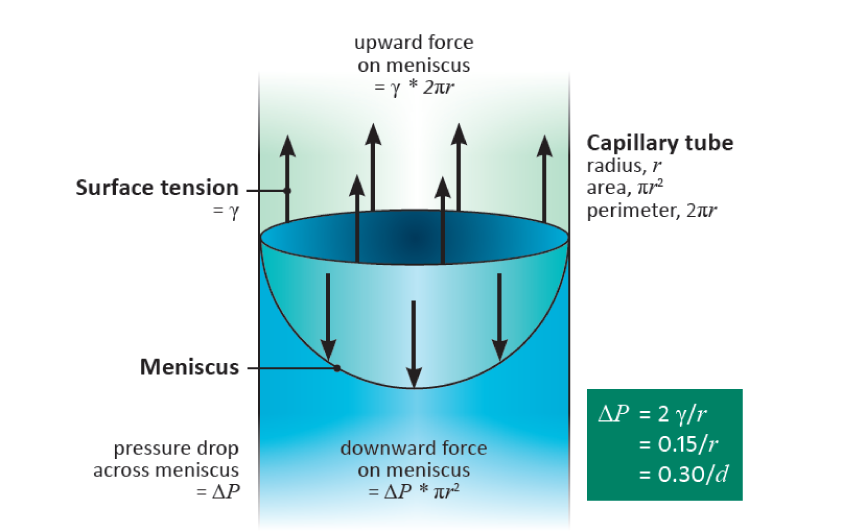# 3.1.3 - Positive and negative hydrostatic pressures

Positive values of hydrostatic pressure occur in the living cells of plants, in the symplast, and as explained above are induced by high solute concentrations and the resultant osmotic pressure. However, large negative values are common in the apoplast of plants and the soil they are growing in. These negative values arise because of capillary effects - the attraction between water and hydrophilic surfaces at an air/water interface, the effects of which can be seen in the way that water wicks into a dry dishcloth. This attraction reduces the pressure in the water, and does so more intensely the narrower are the water-filled pores. It accounts for how cell walls, which have very narrow pores, can remain hydrated despite very low water potentials in the tissue they are part of. For a geometrically simple cylindrical pore, the relation between the induced pressure and the radius of the pore can be derived as follows:

Take a glass capillary tube with a radius $r$ (m) and place it vertically with one end immersed in water. Water will rise in the tube against the gravitational force until an equilibrium is reached at which the weight of the water in the tube is balanced by the force of attraction between the water and the glass. A full, hemispherical, meniscus will have now developed, i.e. one with a radius of curvature equal to that of the tube (Figure 3.6).

## 3.0-Ch-Fig-3.6.pngFigure 3.6 A fully-developed meniscus in a cylindrical tube showing the equality between the upward pull of surface tension and the downward pull of the suction in the water from which the relation $\Delta P = 2\gamma/r$ can be derived.

The meniscus is curved because it is supporting the weight of the water - much as a trampoline sags when several people are sitting on it. There is a difference in pressure, $\Delta P$ (Pa), across the meniscus, with the pressure in the water being less than that of the air. The downward acting force (N) on the meniscus is the difference in pressure multiplied by the cross-sectional area of the tube, i.e $\pi r^2 \Delta P$. The upward acting force is equal to the perimeter of contact between water and glass ($2 \pi r$) multiplied by the surface tension, $\gamma$ (N m-1), of water, namely $2 \pi r \gamma$ (provided the glass is perfectly hydrophilic, when the contact angle between the glass and the water is zero, otherwise this expression has to be multiplied by the cosine of the angle of contact). Thus, because these forces are equal at equilibrium, we have $\pi r^2 \Delta P = 2 \pi r \gamma$, whence

$\Delta P = 2\gamma/r \tag{4}$

The surface tension of water is 0.075 N m-1 at about 20°C, so $\Delta P$ (Pa) equals 0.15 divided by the radius $r$ (m):

$\Delta P = 0.15/r \tag{5}$

Thus a fully-developed meniscus in a cylindrical pore of radius 0.15 mm would have a pressure drop across it of 1.0 MPa. The pressure, $P$, in the water would therefore be -1.0 MPa if referenced to normal atmospheric pressure, or -0.9 MPa absolute pressure (given that standard atmospheric is approximately 100 kPa).

This argument applies not only to cylindrical pores. It is the curvature of the meniscus that determines the pressure drop, and this curvature is uniform over a meniscus occupying a pore of any arbitrary shape. It is such capillary action that generates the low pressures (large suctions) in the cell walls of leaves that induce the long-distance transport of water from the soil through a plant to the sites of evaporation. The pores in cell walls are especially small (diameters of the order of 15 nm), and are therefore able to develop very large suctions, as they do in severely water-stressed plants. Such pores can hold water against a suction of 10 MPa. (Table 3.1)

## 3.0-Ch-Tab-3.1.jpeg

In plants, other water-filled pores vary in size from large xylem vessels with diameters of 100 mm or more down to a few mm, so for them to remain water-filled requires that they have no air/water interfaces.